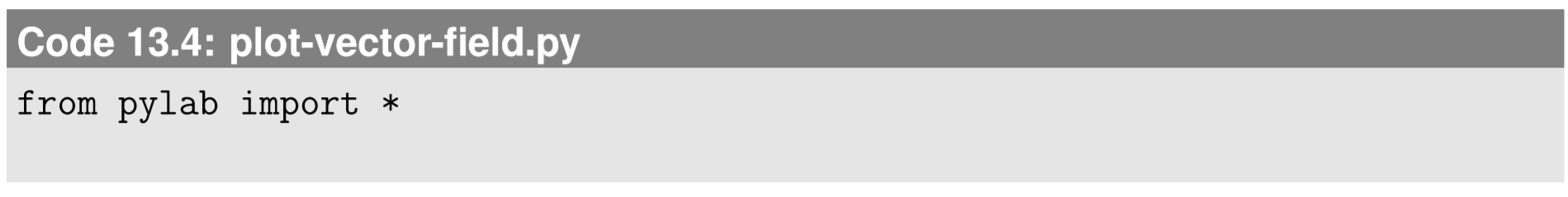$$\newcommand{\id}{\mathrm{id}}$$ $$\newcommand{\Span}{\mathrm{span}}$$ $$\newcommand{\kernel}{\mathrm{null}\,}$$ $$\newcommand{\range}{\mathrm{range}\,}$$ $$\newcommand{\RealPart}{\mathrm{Re}}$$ $$\newcommand{\ImaginaryPart}{\mathrm{Im}}$$ $$\newcommand{\Argument}{\mathrm{Arg}}$$ $$\newcommand{\norm}{\| #1 \|}$$ $$\newcommand{\inner}{\langle #1, #2 \rangle}$$ $$\newcommand{\Span}{\mathrm{span}}$$

13.3: Visualizing Two-Dimensional Scalar and Vector Field

$$\newcommand{\vecs}{\overset { \rightharpoonup} {\mathbf{#1}} }$$

$$\newcommand{\vecd}{\overset{-\!-\!\rightharpoonup}{\vphantom{a}\smash {#1}}}$$

Plotting scalar and vector ﬁelds in Python is straightforward, as long as the space is two-dimensional. Here is an example of how to plot a 3-D surface plot:The scalar ﬁeld $$f(x,y) = \sin{\sqrt{x^2 + y^2}}$$ is given on the right hand side of the zvalues part. The result is shown in Fig. 13.3.1.Figure $$\PageIndex{1}$$: Scalar ﬁeld visualized as a 3-D surface using Code 13.1.

And here is how to draw a contour plot of the same scalar ﬁeld:The clabel command is used here to add labels to the contours. The result is shown in Fig. 13.3.2.Figure $$\PageIndex{2}$$: Scalar ﬁeld visualized as a contour plot using Code 13.2

If you want more color, you can use imshow, which we already used for CA:The result is shown in Fig. 13.3.3. Colorful!Figure $$\PageIndex{3}$$: Scalar ﬁeld visualized as a color image using Code 13.3.

Finally, a two-dimensional vector ﬁeld can be visualized using the streamplot function that we used in Section 7.2. Here is an example of the visualization of a vector ﬁeld v = (vx,vy) = (2x,y−x), with the result shown in Fig. 13.3.4:Figure $$\PageIndex{4}$$: Vector ﬁeld visualized using Code 13.4.

Exercise $$\PageIndex{1}$$:

Plot the scalar ﬁeld $$f(x,y) = \sin{(xy)}$$ for $$−4 ≤ x,y ≤ 4$$ using Python.

Exercise $$\PageIndex{2}$$:

Plot the gradient ﬁeld of f$$(x,y) = \sin{(xy)}$$ for $$−4 ≤ x,y ≤ 4$$ using Python.

Exercise $$\PageIndex{3}$$:

Plot the Laplacian of $$f(x,y) = \sin{(xy)}$$ for $$−4 ≤ x,y ≤ 4$$ using Python. Compare the result with the outputs of the exercises above.